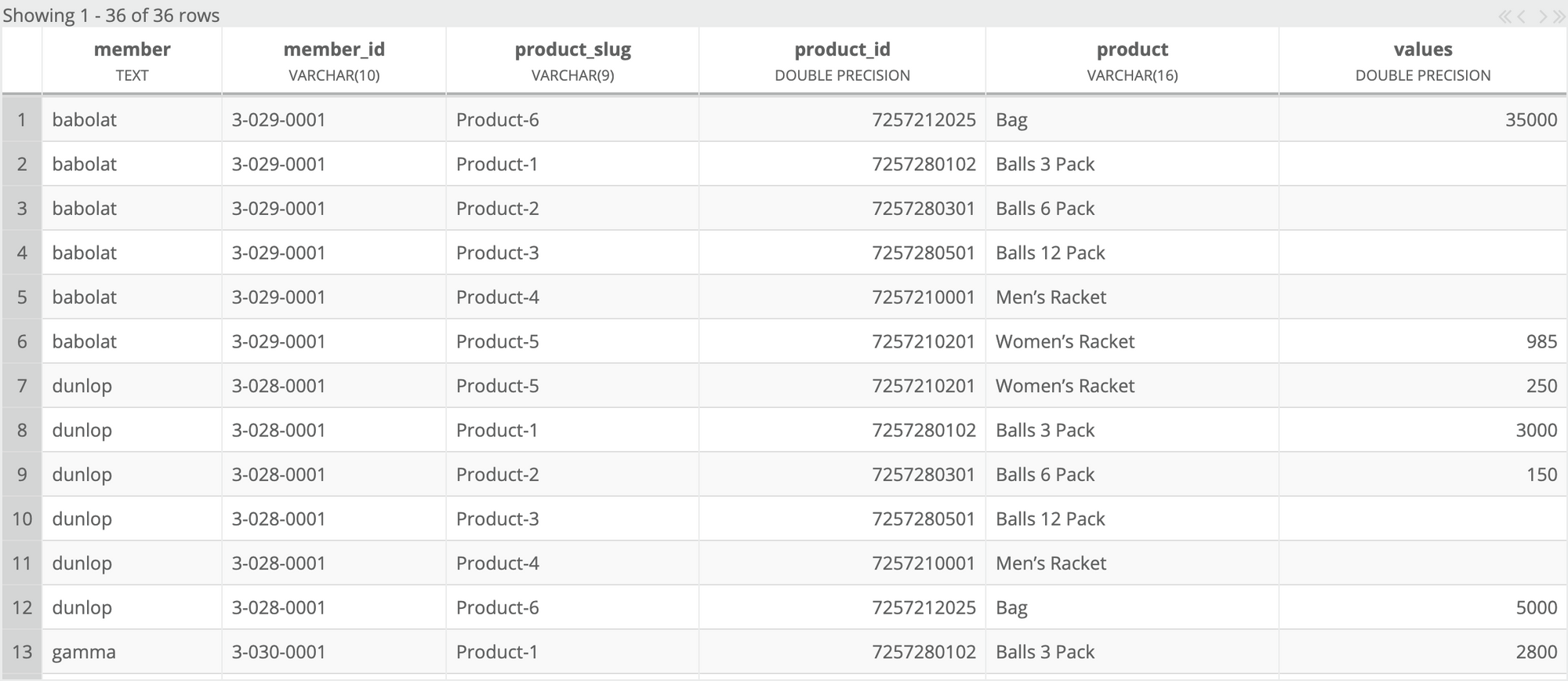# Excel¶

The Excel plugin enables Zuar Runner to pipe data from Excel and store the data in a database.## Create an Excel job¶

Click `+ Add Job`.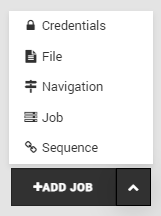Choose `Excel`.Fill out the forms.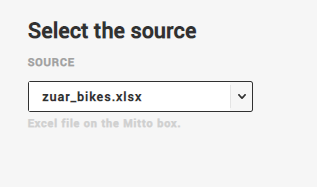• `Source` - Choose the Excel file you want to pipe data from. The Excel file must exist in the Zuar Runner file manager.

Click `Next`.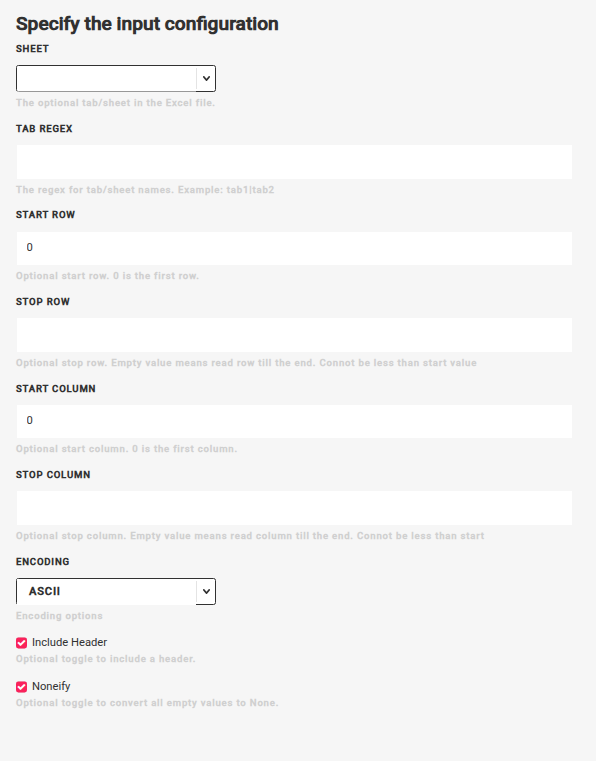• `Sheet` - This is the tab/sheet in the Excel file. You will only create 1 table in the database from 1 sheet in the Excel file. In the Excel plugin wizard, the list of sheets will be available in the dropbox. In the resulting Excel job, `sheet` can either use the name of the sheet as a string or the index of the sheet as an integer (0 is the first sheet).

• `Start Row` - This is optional, but allows you to skip rows in the Excel file. 0 is the first row. 0 by default.

• `Start Column` - This is optional, but allows you to skip columns in the Excel file. 0 is the first column. 0 by default.

• `Encoding` - This allows you to select the encoding used on the excel file.

• `Include Header` - Check this if the data has a header. Checked by Default.

• `Noneify` - Check this if you want to convert empty values to NULL. Checked by default.

Click `Next`.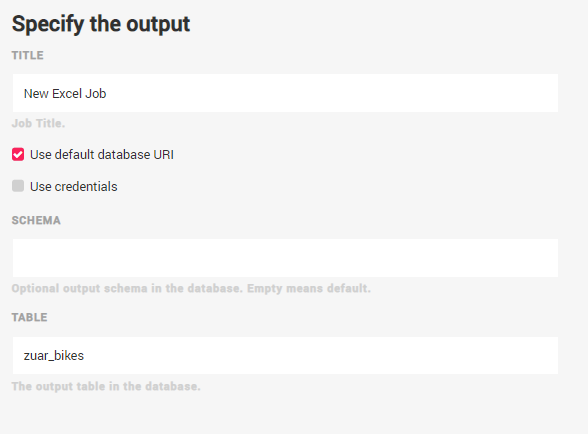• `Title` - This is the resulting job’s title.

• `Use default database URL` - Select this to use the local database. Unselect to enter an external database URL.

• `Use Credentials` - Select this to use credentials

• `Table` - This is the output table.

• `Schema` - This is the output schema.

For Excel job naming best practices, see the following convention:

• If the name of the Excel file is `Orders.xlsx` and the name of the tab in the file is `Sheet1` then the name of the job should be: `[Excel] Orders.xlsx - Sheet 1`.

Click `Done`.

## Configure an Existing Excel Job¶

Navigate to the job and click `Edit`.The main sections to edit are `input` and `output`.

### Input:¶

`source`: This is the Excel file on the Zuar Runner box.

`sheet`: This is the tab/sheet in the Excel file. You will only create 1 table in the database from 1 sheet in the Excel file. `sheet` can either use the name of the sheet as a string or the index of the sheet as an integer (0 is the first sheet).

`start_column`: This is optional, but allows you to skip columns in the Excel file. 0 is the first column.

`start_row`: This is optional, but allows you to skip rows in the Excel file. 0 is the first row.

`encoding`: This is required and tells Zuar Runner how the excel sheet is encoded.

`include_headers`: This is required and tells Zuar Runner whether or not to include the header row of the sheet.

`noneify`: This is required and tells Zuar Runner how to handle `Null` values in the sheet.

`use`: This is required and specifies the underlying python input script to be used when inputting the sheet.

### Output:¶

`dbo`: This is the connection string of the output database. If you are outputting data into Zuar Runner’s internal PostgreSQL database, leave this as is.

`schema`: This is the output schema in the database.

`table`: This is the output table in the database.

`use`: This is the underlying python output script to be used when sending the data from the sheet to a relational database table.

## Use Case: How to Manipulate Excel Data for Analytics¶

Let’s pretend that you need to pull a subset of data from an Excel spreadsheet, and pivot that Excel data to create a new table with a specific data structure.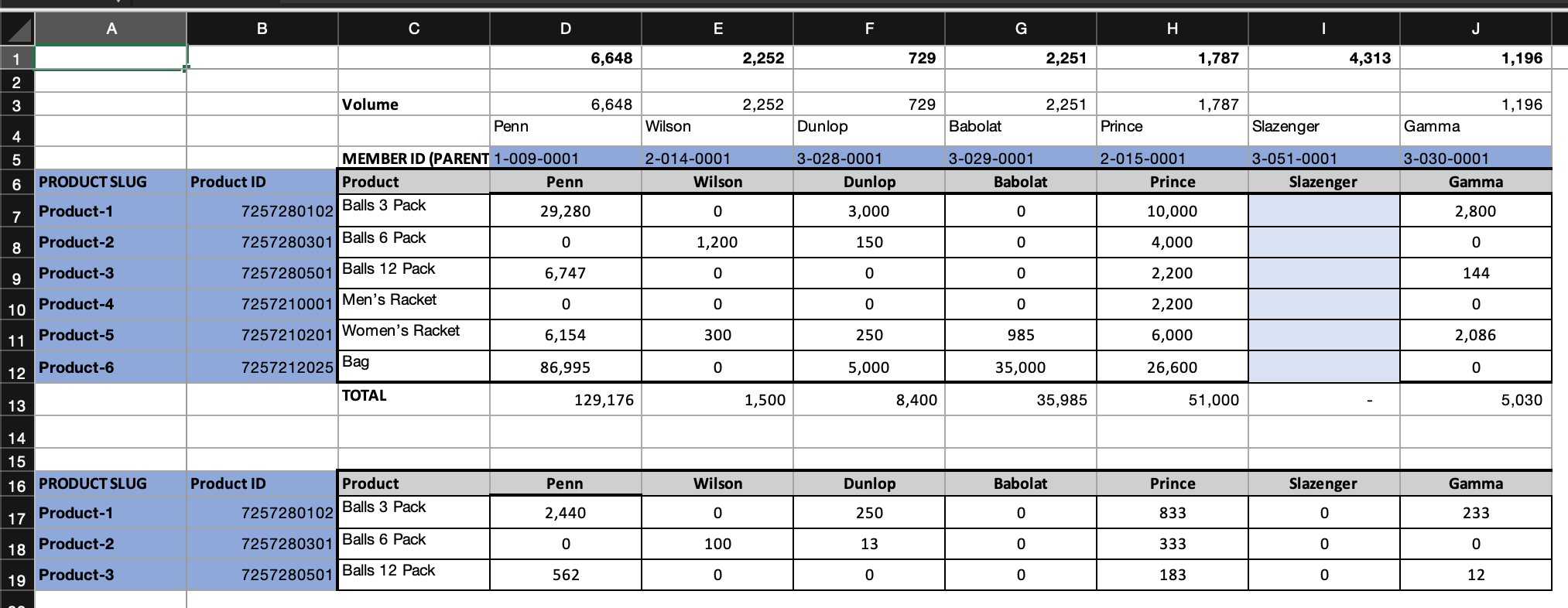You want a final table with Member, Member ID, Product SLUG, Product ID, Product and all of the product volume data (cells C7-J12). You also only want data from the first table in the Excel sheet and do not want the total rows (row 13).

First, you need to pull two sets of data from the spreadsheet. One set will have member, and the member ID (in green below), and the other set will include all the product data (in red below).

Next, you need to pivot that data into a vertical format. Finally, you will do a full outer join on the two pivoted tables, and then run everything in sequence.

### Excel Jobs in Zuar Runner¶

In Zuar Runner, you’ll create two Excel jobs. Both Excel jobs use the same Excel file as a data source, but each job will grab a different section of Excel data.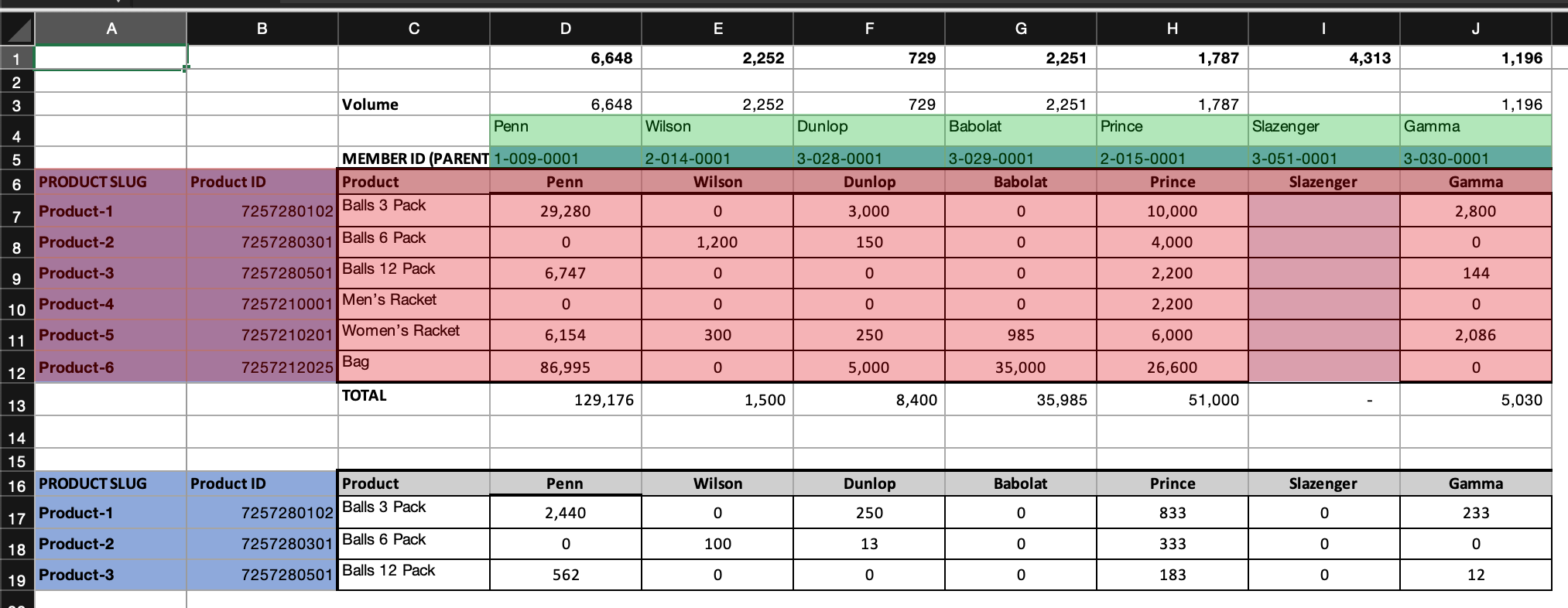The first Excel job (in green) will have a start column of 3 and a start row of 3 (always using a zero index), and should only include 2 rows.

The second job (in red) will start at row 5 and should only include 7 rows. `start_column` and `start_row` are keys that can be provided in the “input” block of a job. To stop pulling data after a set number of rows we can use the `SliceTransform` class in the “transforms” step.

Consider the following JSON for the first job (in green):

```{
"input": {
"sheet": "Sheet 1",
"source": "Blog_Example.xlsx",
"start_column": 3,
"start_row": 3,
"use": "flatfile.iov2#ExcelInput"
},
"output": {
"dbo": "postgresql://db/analytics",
"schema": "excel",
"tablename": "pivot_example_blog_members",
"use": "call:mitto.iov2.db#todb"
},
"steps": [
{
"transforms": [
{
"stop": 1,
"use": "mitto.iov2.transform.builtin#SliceTransform"
},
{
"use": "mitto.iov2.transform#ExtraColumnsTransform"
},
{
"use": "mitto.iov2.transform#ColumnsTransform"
}
],
"use": "mitto.iov2.steps#Input"
},
{
"use": "mitto.iov2.steps#CreateTable"
},
{
"transforms": [
{
"use": "mitto.iov2.transform#FlattenTransform"
}
],
"use": "mitto.iov2.steps#Output"
},
{
"use": "mitto.iov2.steps#CountTable"
}
]
}
```

This is the JSON defining the first job (in green). You will note there are 3 blocks, “input,” “output,” and “steps.”

At the top in the “input” block, you will see the `start_row` and the `start_columns` defined. Zuar Runner always uses zero indexes for tabular data rows and columns, meaning we start counting at zero, not one. Therefore, the fourth cell from the left is `"start_column": 3`.

The second block, “output,” defines where the resulting data will be stored. This could define anything, including flat files, but in this case we’re outputting the Excel data into Zuar Runner’s built in PostgreSQL database in a schema named `excel`, and a table named `pivot_example_blog_members`.

In the “steps” block, we define each step of transforming the data from Excel to SQL, including the very first step `SliceTransform`, which limits the number of rows we want from the spreadsheet to one. Again starting at a zero index, so `1` will yield two rows.

Running this job will produce the following table:

Running a similar job for the data in red will produce this table: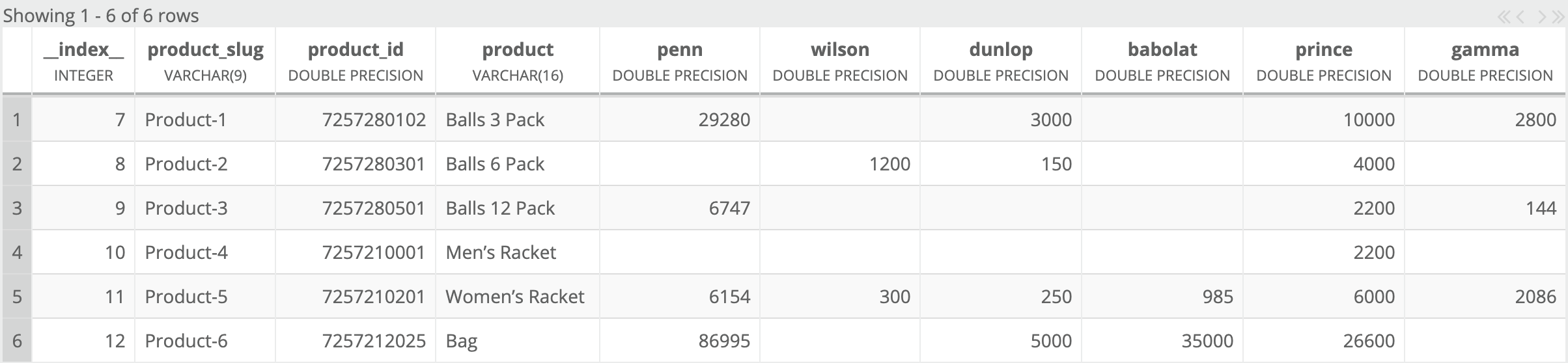### SQL Jobs¶

Now that you have Excel data in the database, you need to transform the data to get to the final result the user wants.

You’ll create two more Zuar Runner jobs in order to pivot the data from horizontal to vertical. These jobs will be SQL jobs using PostgreSQL syntax. In order to pivot the data you’ll use the LATERAL keyword, which is kind of like an SQL for-loop. For every product in our table we want to list each member and their volume data. The SQL is as follows:

```DROP TABLE IF EXISTS excel.pivotted_table;
CREATE TABLE IF NOT EXISTS excel.pivotted_table AS
SELECT
t.__index__
, t.product_slug
, t.product_id
, t.product
, v.*
FROM
excel.pivot_examples_blog_table t
, LATERAL (VALUES
('penn', t.penn)
, ('wilson', t.wilson)
, ('dunlop', t.dunlop)
, ('babolat', t.babolat)
, ('prince', t.prince)
, ('gamma', t.gamma)
) v (member, values)
;
```

For the member pivot, we’ll use:

```DROP TABLE IF EXISTS excel.pivotted_members;
CREATE TABLE IF NOT EXISTS excel.pivotted_members AS
SELECT
t.__index__
, v.*
FROM
excel.pivot_example_blog_members t
, LATERAL (VALUES
('penn', t.penn),
('wilson', t.wilson),
('dunlop', t.dunlop),
('babolat', t.babolat),
('prince', t.prince),
('gamma', t.gamma)) v (member, member_id)
;
```

The resulting tables will look like this:

One final SQL job will do a full outer join on these two tables on the member column:

```DROP TABLE IF EXISTS excel.joined_pivot;
CREATE TABLE IF NOT EXISTS excel.joined_pivot AS
SELECT
excel.pivotted_members.member
, excel.pivotted_members.member_id
, excel.pivotted_table.product_slug
, excel.pivotted_table.product_id
, excel.pivotted_table.product
, excel.pivotted_table.values
FROM
excel.pivotted_members
FULL OUTER JOIN excel.pivotted_table
ON excel.pivotted_members.member = excel.pivotted_table.member
;
```

### Sequence all Jobs Together to Manipulate Excel Data¶

Finally in Zuar Runner, click the up arrow next to the “Add” button and select “Sequence.” In the order they were made, drag all the jobs one by one into the box on the left, and make sure each job has “Enabled” checked. Click on “Submit” to create the sequence and then run it.

If all went well you should end up with a table like this: